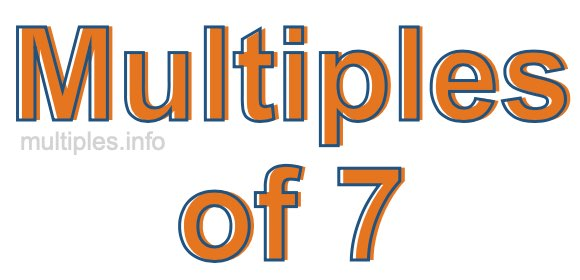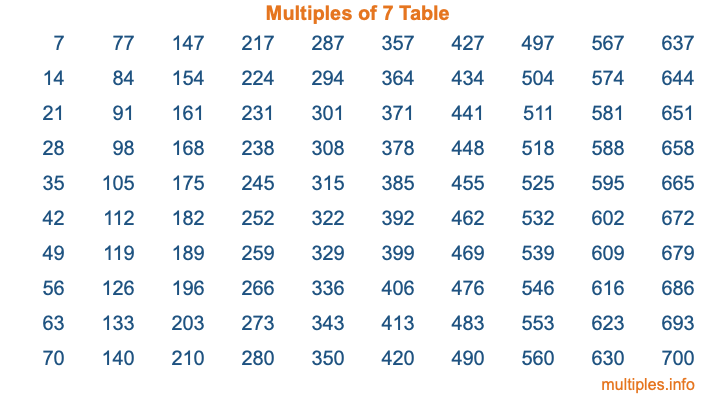Multiples of 7Welcome to the Multiples of 7 page. Here we will first teach you everything you will ever need to know about the multiples of 7, and then give you a study guide summary of everything we taught you to make sure you remember it all. Use this page to look up facts and learn information about the multiples of 7. This page will make you a multiples of seven expert!

Definition of Multiples of 7
Multiples of 7 are all the numbers that when divided by 7 equal an integer. Each of the multiples of 7 are called a multiple. A multiple of 7 is created by multiplying 7 by an integer.

Therefore, to create a list of multiples of 7, you start with 1 multiplied by 7, then 2 multiplied by 7, then 3 multiplied by 7, and so on for as long as you want. Thus, the list of the first five multiples of 7 is 7, 14, 21, 28, and 35. To see a larger list of multiples of 7, see the printable image of Multiples of 7 further down on this page. We also have a category where you can choose any nth multiple of 7.

Multiples of 7 Checker
The Multiples of 7 Checker below checks to see if any number of your choice is a multiple of 7. In other words, it checks to see if there is any number (integer) that when multiplied by 7 will equal your number. To do that, we divide your number by 7. If the the quotient is an integer, then your number is a multiple of 7.

Is  a multiple of 7?

Least Common Multiple of 7 and ...
A Least Common Multiple (LCM) is the lowest multiple that two or more numbers have in common. This is also called the smallest common multiple or lowest common multiple and is useful to know when you are adding our subtracting fractions. Enter one or more numbers below (7 is already entered) to find the LCM.

Check out our LCM Calculator if you need more details about the Least Common Multiple or if you need the LCM for different numbers for adding and subtraction fractions.

nth Multiple of 7
As we stated above, 7 is the first multiple of 7, 14 is the second multiple of 7, 21 is the third multiple of 7, and so on. Enter a number below to find the nth multiple of 7.

th multiple of 7

Multiples of 7 vs Factors of 7
7 is a multiple of 7 and a factor of 7, but that is where the similarities end. All postive multiples of 7 are 7 or greater than 7. All positive factors of 7 are 7 or less than 7.

Below is the beginning list of multiples of 7 and the factors of 7 so you can compare:

Multiples of 7: 7, 14, 21, 28, 35, etc.

Factors of 7: 1, 7

As you can see, the multiples of 7 are all the numbers that you can divide by 7 to get a whole number. The factors of 7, on the other hand, are all the whole numbers that you can multiply by another whole number to get 7.

It's also interesting to note that if a number (x) is a factor of 7, then 7 will also be a multiple of that number (x).

Multiples of 7 vs Divisors of 7
The divisors of 7 are all the integers that 7 can be divided by evenly. Below is a list of the divisors of 7.

Divisors of 7: 1, 7

The interesting thing to note here is that if you take any multiple of 7 and divide it by a divisor of 7, you will see that the quotient is an integer.

Multiples of 7 Table
Below is an image of the first 100 multiples of 7 in a table. The table is in chronological order, column by column. The first column has the first ten multiples of 7, the second column has the next ten multiples of 7, and so on.The Multiples of 7 Table is also referred to as the 7 Times Table or Times Table of 7. You are welcome to print out our table for your studies.

Negative Multiples of 7
Although not often discussed or needed in math, it is worth mentioning that you can make a list of negative multiples of 7 by multiplying 7 by -1, then by -2, then by -3, and so on, to get the following list of negative multiples of 7:

-7, -14, -21, -28, -35, etc.

Multiples of 7 Summary
Below is a summary of important Multiples of 7 facts that we have discussed on this page. To retain the knowledge on this page, we recommend that you read through the summary and explain to yourself or a study partner why they hold true.

There are an infinite number of multiples of 7.

A multiple of 7 divided by 7 will equal a whole number.

7 divided by a factor of 7 equals a divisor of 7.

The nth multiple of 7 is n times 7.

The largest factor of 7 is equal to the first positive multiple of 7.

7 is a multiple of every factor of 7.

7 is a multiple of 7.

A multiple of 7 divided by a divisor of 7 equals an integer.

7 divided by a divisor of 7 equals a factor of 7.

Any integer times 7 will equal a multiple of 7.

Multiples of a Number
Here you can get the multiples of another number, all with the same attention to detail as we did for multiples of 7 on this page.

Multiples of
Multiples of 8
Did you find our page about multiples of seven educational? Do you want more knowledge? Check out the multiples of the next number on our list!